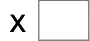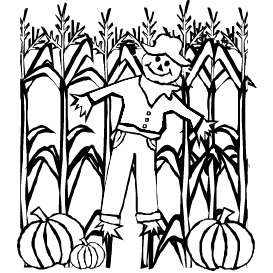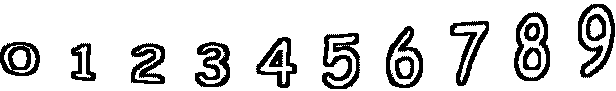8= 56   5= 457= 35   8= 80   6= 36   4= 4 6= 6   3= 18   2= 12   3= 12